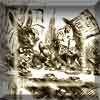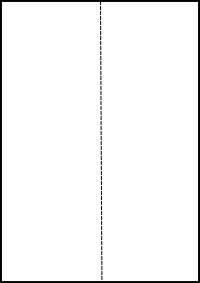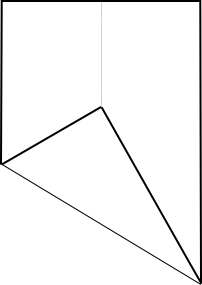You may also likeA Problem of Time

Consider a watch face which has identical hands and identical marks for the hours. It is opposite to a mirror. When is the time as read direct and in the mirror exactly the same between 6 and 7?The points P, Q, R and S are the midpoints of the edges of a non-convex quadrilateral.What do you notice about the quadrilateral PQRS and its area?

Making Sixty

Age 14 to 16Challenge Level

Take a piece of paper.Fold it along the long axis and then open it up.Now fold one corner over and onto the centre crease so that the fold line passes through the corner next to it (on the short side of the paper).

You have created some angles. There are angles of $60^o$ and of $30^o$. Can you prove this?

Does the paper have to be A4?

You are now able to fold the paper to make an equilateral triangle which can be used in lots of different ways. For example, see Equilateral Triangle Folding .